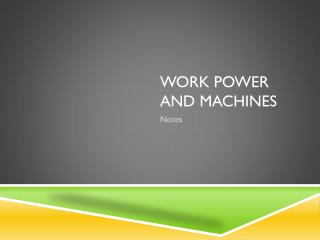Download PresentationWork Power and Machines

# Work Power and Machines

Download Presentation## Work Power and Machines

- - - - - - - - - - - - - - - - - - - - - - - - - - - E N D - - - - - - - - - - - - - - - - - - - - - - - - - - -
##### Presentation Transcript

1. Work • The transfer of energy to an object when a force makes it go in the direction of the force.

2. Work is Done When… • An object gets kinetic energy from an applied force • An object moves in the same direction as the force • Only when force is applied

3. Work is not done when… • An object does not move despite force applied • An object moves but in a different direction than the force

4. The Amount of Work Done… • W = F * D (work = force times distance) • Unit of measure = Joules (J) • Is the same if: • driving up a road (less force, more distance) and • straight up a cliff (more force, less distance)

5. Power = the rate of work • P = W / T (power equals work divided by time) • Unit of measure is Watts…(Joules per second) • If work is done faster then more power is used… • sanding wood by hand vs. using a power tool